Next: Basic Equations for Radiative Up: Hydrostatic Equilibrium Previous: Polytrope   Contents

# Magnetohydrostatic Configuration

In section 4.2, we obtained the maximum mass which is supported against the self-gravity using the virial analysis. In this section, we will survey result of more realistic calculation.

Formalism was obtained by Mouschovias (1976a,6), which was extended by Tomisaka, Ikeuchi, & Nakamura (1988) to include the effect of rotation. Magnetohydrostatic equlibrium is achived on a balance between the Lorentz force, gravity, thermal pressure force, and the centrifugal force as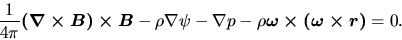(C.13)

In the axisymmetric case, the poloidal magnetic fields is obtained by the magnetic flux function,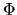, or the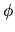-component of the vector potential as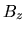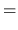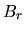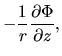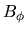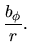(C.14)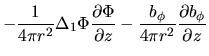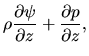(C.15)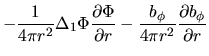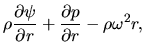(C.16)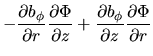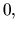(C.17)

with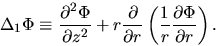(C.18)

Equation (C.17) indicates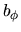is a function ofas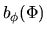, which is constant along one magnetic field line. Ferraro's isorotation law demands, that is, to satisfy the stead-state induction equation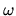is constant along a magnetic field. This meansis also constant along one magnetic field line,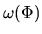. From this, the density distribution in one flux tube is written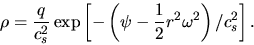(C.19)

This means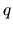is also constant along one magnetic field line,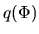. Since the forces are expressed by the defrivative of function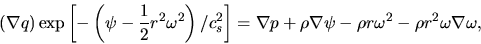(C.20)

where equation (C.19) is used, equation (C.15) and (C.16) are rewritten as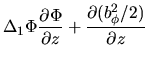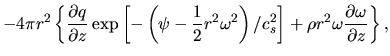(C.21)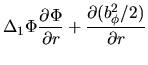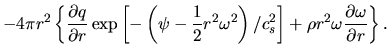(C.22)

Finally, using the fact that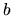,, andare functions of, these two equations are reduced to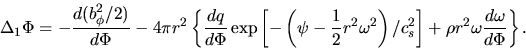(C.23)

Another equation to be coupled is the Poisson equation as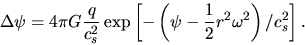(C.24)

The source terms of equations (C.23) and (C.24) are given by determining the mass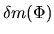and the angular momentum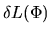contained in a flux tube-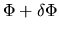. Mass and angular momentum distribution of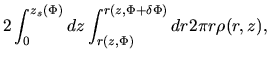(C.25)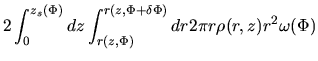(C.26)

is chosen artitrary in nature, where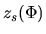is the the height of the cloud surface where the magnetic potential is equal to. For example,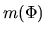and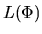are chosen as a uniformly rorating uniform-density spherical cloud threaded by uniform magnetic field. Since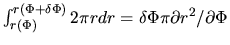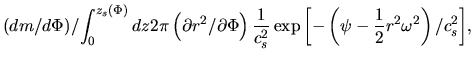(C.27)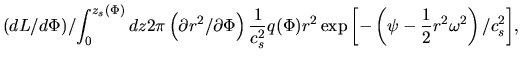(C.28)

The source terms of PDEs [eqs (C.23) and (C.24)] are given from equations (C.27) and (C.28). While the functonsandare determined from the solution of these PDEs afterandare chosen. This can be solved by a self-consistent field method.Next: Basic Equations for Radiative Up: Hydrostatic Equilibrium Previous: Polytrope   Contents
Kohji Tomisaka 2007-07-08